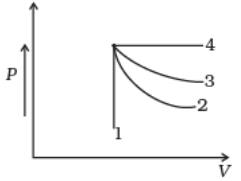Courses

# Test: Heat Engines

## 10 Questions MCQ Test Physics Class 11 | Test: Heat Engines

Description
This mock test of Test: Heat Engines for JEE helps you for every JEE entrance exam. This contains 10 Multiple Choice Questions for JEE Test: Heat Engines (mcq) to study with solutions a complete question bank. The solved questions answers in this Test: Heat Engines quiz give you a good mix of easy questions and tough questions. JEE students definitely take this Test: Heat Engines exercise for a better result in the exam. You can find other Test: Heat Engines extra questions, long questions & short questions for JEE on EduRev as well by searching above.
QUESTION: 1

### In a heat engine the ratio of net work done per cycle by the engine to the total amount of heat absorbed per cycle by the working substance from the source is known as

Solution:

In a heat engine the ratio of net work done per cycle by the engine to the total amount of heat absorbed per cycle by the working substance from the source is known as Thermal Efficiency.

QUESTION: 2

### In actual home refrigerator vapours of Freon ( which is dichlorodifluoro methane CCl2F2) act as

Solution:

Dichlorodifluoromethane (R-12) is a colorless gas usually sold under the brand name Freon-12, and a chlorofluorocarbon halomethane (CFC) used as a refrigerant and aerosol spray propellant. Complying with the Montreal Protocol, its manufacture was banned in developed countries (non-article 5 countries) in 1996, and developing countries (article 5 countries) in 2010 due to concerns about its damaging impact to the ozone layer.[better source needed] Its only allowed usage is as fire retardant in submarines and aircraft. It is soluble in many organic solvents. Dichlorodifluoromethane was one of the original propellants for Silly String. R-12 cylinders are colored white.

QUESTION: 3

### A carnot engine is taking 700 cal from source and is rejecting 500 cal to the sink in each cycle. What is the temperature of sink if the source temperature is 150° C .

Solution:

Heat extracted from source Q1= 700 cal
Heat rejected to the sink Q2= 500 cal
Temperature of source T1= 150 +273= 423K
Temperature of sink T2 to be found:
Q2/Q1 = T2/T1
So,
T2 = (Q2/Q1) x T1
= (500 x 423)/700
= 302.02 K
= 29.02 degree C.

QUESTION: 4

According to Carnot theorem no heat engine working between two given temperatures of source and sink can be more efficient than a perfectly ___________ engine working between the same two temperatures

Solution:

According to Carnot theorem no heat engine working between two given temperatures of source and sink can be more efficient than a perfectly reversible engine working between the same two temperatures, by the statement of Carnot theorem.

QUESTION: 5

How much heat energy a petrol engine would require to do 20 kWh of work? Its adiabatic compression ratio is 9 and γ= 1.5.

Solution:
QUESTION: 6

An ideal gas undergoes four different processes from the same initial state (Fig.). Four processes are adiabatic, isothermal, isobaric and isochoric. Out of 1, 2, 3 and 4 which one is adiabatic.Solution:

4 is isobaric process, 1 is isochoric. Out of 3 and 2, 3 has the smaller slope (magnitude) hence is isothermal. Remaining process 2 is adiabatic.

QUESTION: 7

What would be the horse power of a steam engine with average pressure of steam 9x 104 Nm-2 , the area of cross section of the piston is 0.2 m2, length of stroke is 0.6 m and piston makes 5 revolutions per second?

Solution:

Energy in 1 revolution=2 x P x a x l=2 x 9 x104 x 0.2 x0.6 j=21600J
Energy in n revolutions= 21600nJ
Power=E/t=21600n/t=21600 x 5=18000 J/s= 108000/746 h.p=144.77 h.p.

QUESTION: 8

The ratio of quantity of heat removed per cycle from the contents of the refrigerator to the energy spent per cycle to remove this heat is called the

Solution:

The ratio of quantity of heat removed per cycle from the contents of the refrigerator to the energy spent per cycle to remove this heat is called the coefficient of performance. It is the definition of coefficient of performance.

QUESTION: 9

In a Carnot engine 800 J of heat is absorbed from a source at 400 K and 640 J of heat is rejected to the sink. The temperature of the sink is:

Solution:

for hot reservoir I.e. source
temperature T1=400k
heat supplied Q1=800J
For cold reservoir i.e. sink
Temperature T2=?
Heat supplied Q2=640J
According to carnot’s theorem,
Q1/Q2= T2/T1
640/800= T2/400
T2=320k

QUESTION: 10

The temperature inside a refrigerator is 4°C and the room temperature is 27°C. How many joules of heat will be delivered to the room for each joule of electricity consumed by the refrigerator?( Treat the refrigerator as ideal).

Solution:

Coefficient of performance(cop) of a refrigerator,
β= Q2/Q1-Q2=T2/T1-T2=277/300-277=277/23≈12
OR, Q2/W=12. Therefore, W=1J.Q2=12J
Also, Q1=Q2+W
=(12+1) J
=13J